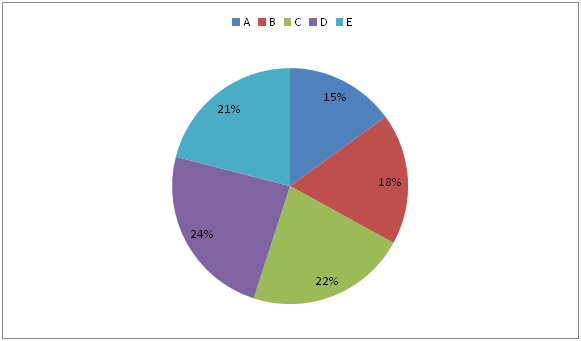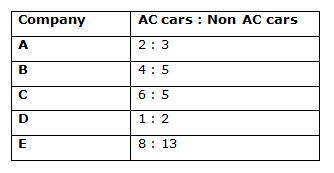# Quantitative Aptitude Questions (Data Interpretation) for IBPS RRB Assistant Prelims 2018 Day-168

Dear Readers, IBPS RRB is conducting Online Examination for the recruitment of clerical cadre To enrich your preparation here we have providing new series of Data Interpretation – Quantitative Aptitude Questions. Candidates those who are appearing in IBPS RRB Assistant Prelims Exams can practice these Quantitative Aptitude average questions daily and make your preparation effective.

Click “Start Quiz” to attend these Questions and view Solutions

Directions (1 – 5): Study the following information carefully and answer the given questions:

There are two trains A and B in a platform. Some passengers didn’t buy any tickets. Total number of passengers travelling with ticket in both trains is 3000. The ratio between the total number of passengers buy a ticket travelled in train A to that of train B is 3: 2. The ticketless passengers in both the trains are same, which is equal to 150.

1) Total number of passengers travelled in Train A is?

a) 1560

b) 1720

c) 1950

d) 1840

e) None of these

2) If the ratio between the male and female in train B is 2 : 1, then find the ratio between the total number of female in train B to that of total number of passengers didn’t buy any tickets in train A?

a) 3: 1

b) 5: 3

c) 2: 5

d) 4: 7

e) None of these

3) If 18 % of the passengers in train A bought AC tickets, then find the total number of passengers bought other than AC tickets in train A?

a) 1352

b) 1278

c) 1154

d) 1476

e) None of these

4) Find the difference between the total number of passengers travelled in train A to that of train B?

a) 750

b) 600

c) 500

d) 450

e) None of these

5) Find the approximate percentage of total number of ticketless passengers out of the total number of passengers in train B?

a) 10 %

b) 25 %

c) 35 %

d) 50 %

e) 20 %

Directions (6 – 10): Study the following information carefully and answer the given questions:

Following pie chart shows the total number of cars sold by five different companies in a certain year.

Total number of cars sold = 25 lakhsThe table shows the ratio of total number of AC cars and Non AC cars sold by these five companies.6) Total number of Non AC cars sold by Company B is approximately what percentage of total number of AC cars sold by Company C?

a) 75 %

b) 85 %

c) 105 %

d) 50 %

e) 65 %

7) Find the ratio between the total number of AC cars sold by Company D and E together to that of total number of cars sold by Company B?

a) 6: 7

b) 5: 6

c) 2: 3

d) 8: 9

e) None of these

8) Find the total number of AC cars sold by Company A, C and D together?

a) 5.6 lakhs

b) 4.7 lakhs

c) 6.5 lakhs

d) 7.2 lakhs

e) None of these

9) Find the difference between the total number of cars sold by Company A to that of Company D?

a) 2.25 lakhs

b) 2.5 lakhs

c) 1.75 lakhs

d) 1.5 lakhs

e) None of these

10) Total number of cars sold by Company A is approximately what percentage less than the total number of cars sold by Company E?

a) 55 %

b) 15 %

c) 5 %

d) 30 %

e) 45 %

Total number of ticketless passengers in train A = 150

Total number of passengers travelling with a ticket in train A

= > 3000*(3/5) = 1800

Total number of passengers travelled in train A = 1800 + 150 = 1950

The ratio between the male and female in train B = 2: 1 (2x, x)

Total number of ticketless passengers in train A = 150

Total number of ticketless passengers in train B = 150

Total numbers of passengers travelling with a ticket in train B

= > 3000*(2/5) = 1200

Total number of passengers travelled in train B = 1200 + 150 = 1350

The total number of female in train B = 1350*(1/3) = 450

Required ratio = 450: 150 = 3: 1

Total number of passengers travelling with a ticket in train A

= > 3000*(3/5) = 1800

The total number of passengers bought other than AC tickets in train A

= > 1800*(82/100) = 1476

Total number of ticketless passengers in train A = 150

Total number of passengers travelling with a ticket in train A

= > 3000*(3/5) = 1800

Total number of passengers travelled in train A = 1800 + 150 = 1950

Total number of ticketless passengers in train B = 150

Total numbers of passengers travelling with a ticket in train B

= > 3000*(2/5) = 1200

Total number of passengers travelled in train B = 1200 + 150 = 1350

Required difference = 1950 – 1350 = 600

Total number of ticketless passengers in train B = 150

Total numbers of passengers travelling with a ticket in train B

= > 3000*(2/5) = 1200

Total number of passengers travelled in train B = 1200 + 150 = 1350

Required % = (150/1350)*100 = 11.11 % = 10 %

Total number of Non AC cars sold by Company B

= > 25*(18/100)*(5/9) = 2.5 lakhs

Total number of AC cars sold by Company C

= > 25*(22/100)*(6/11) = 3 lakhs

Required % = (2.5/3)*100 = 83.33 = 85 %

The total number of AC cars sold by Company D and E together

= > 25*(24/100)*(1/3) + 25*(21/100)*(8/21)

= > 2 + 2 = 4 lakhs

The total number of cars sold by Company B

= > 25*(18/100) = 4.5 lakhs

Required ratio = 4: 4.5 = 40 : 45 = 8 : 9

The total number of AC cars sold by Company A, C and D together

= > 25*(15/100)*(2/5) + 25*(22/100)*(6/11) + 25*(24/100)*(1/3)

= > 1.5 + 3 + 2 = 6.5 lakhs

The total number of cars sold by Company A

= > 25*(15/100) = 3.75 lakhs

The total number of cars sold by Company D

= > 25*(24/100) = 6 lakhs

Required difference = 6 – 3.75 = 2.25 lakhs

Required % = [(21- 15)/21]*100 = 30 %

Daily Practice Test Schedule | Good Luck

 Topic Daily Publishing Time Daily News Papers & Editorials 8.00 AM Current Affairs Quiz 9.00 AM Quantitative Aptitude “20-20” 11.00 AM Vocabulary (Based on The Hindu) 12.00 PM General Awareness “20-20” 1.00 PM English Language “20-20” 2.00 PM Reasoning Puzzles & Seating 4.00 PM Daily Current Affairs Updates 5.00 PM Data Interpretation / Application Sums (Topic Wise) 6.00 PM Reasoning Ability “20-20” 7.00 PM English Language (New Pattern Questions) 8.00 PM